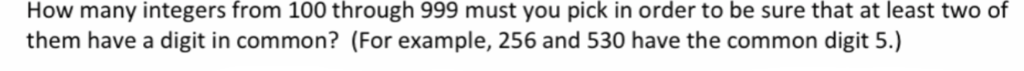# Solved: How Many Integers From 100 Through 999 Must You Pick In Order To Be Sure That At Least Two Of Them Have A Digit In Common? (For Example, 256 And 530 Have The Common Digit 5.)

By |Discreet Math / Probability,

Show clear steps

How many integers from 100 through 999 must you pick in order to be sure that at least two of them have a digit in common? (For example, 256 and 530 have the common digit 5.)